Are you more of a visual learner? Check out our online video lectures and start your chemistry course now for free!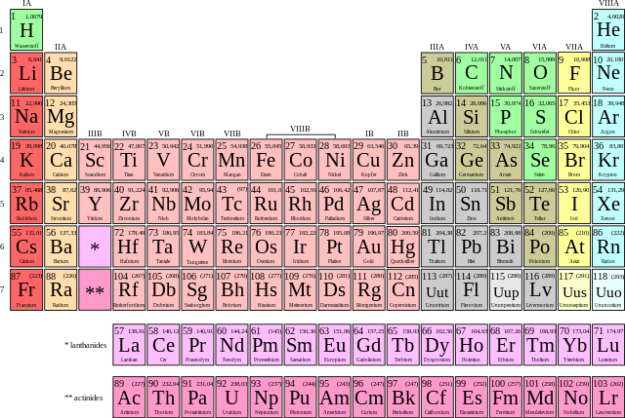Image: “ Periodensystem der Elemente” by Saehrimnir. License: CC BY-SA 3.0

## The Atom and Atomic Structure

Atoms are the basic units of all matter. They consist of a positively charged nucleus and a negatively charged shell in which the electrons move in fixed, elliptical orbits.

Atoms are made of three fundamental particles:

 Charge Mass/ amu Nucleons Protons +1 1.0073 Neutrons 0 1.0087 Electrons -1 5.4859 x 10-4
1 atomic mass unit (amu) = 1.6605 x 10-24 g

Atoms and elements are defined by three numbers relating to the composition of the nucleus:

• Z – the ATOMIC (or ELEMENT) number
• N – the NEUTRON number
• A – the MASS number

To properly identify an atom, the chemical name is written as follows:### Atomic core

The atomic core (nucleus) possesses positively charged protons and neutrons, which have no charge. What’s special about the nucleus? Compared to the electron shell, it is much smaller, but yet it accounts for 99% of the mass of the whole atom.

### Nuclide and nucleons

The nuclide is a particular type of nucleus, which is precisely defined by the number of specific nucleons inside it. Nucleons are a collective term used to refer to subatomic particles inside the nucleus, which are the protons and the neutrons. Nuclides that have the same number of protons, but a different number of neutrons, are referred to as isotopes of that element.

### Isotopes

Isotopes represent atoms of the same element that differ in the number of neutrons. If the mass number in the periodic table of elements represents a fraction, the naturally occurring isotopes will be taken into account as a percentage. To calculate the mass number, the following equation is used.

Example: Carbon has an atomic number of 6 (which means each carbon atom has 6 protons and 6 neutrons) and a mass number of 12.011:

• 6 + 6 = 12 → According to the mass number, there is a difference of 0.011.
• Conclusion: In some atoms of the element carbon, there are different numbers of neutrons, and these atoms are known as isotopes.

For approximately 20 of the mononuclidic elements (including sodium, phosphorus, and fluorine), only one stable isotope exists; these are called monoisotopic elements.

Mixed elements are elements that contain different stable isotopes, such as hydrogen, which contains the stable isotopes protium and tritium.

### Shells

In the shells of the atom, the electrons move in elliptical orbits around the nucleus. The electrons fill the shells from the inside to the outside. These shells are named in alphabetical order, starting with the letter K. This means that the first or the innermost shell is called “K,” the second one “L,” and the third one “M,” etc.

### Ions

Atoms are neutral-charged particles, which means that they possess equal numbers of electrons and protons. If an atom releases an electron as part of a reaction and incorporates other electrons, then it is called a charged atom, which is known as an ion. Positive ions, which are ions with fewer electrons than protons, are referred to as cations. Negative ions, which are ions with an excess of electrons, are known as anions.

Ions of the main elements follow the octet rule to attain noble gas configuration.

## The Periodic System of Elements (PSE)

### Ordering principle

The periodic table is arranged based on the atomic structure of the elements, reflecting their structure and properties while also displaying other values, such as electronegativity.

The periodic table is read from top to bottom and from left to right, following a sequence of increasing atomic numbers. The atomic number represents the number of protons an atom of a particular element possesses.

The periodic table is called as such because the elements are horizontally divided into rows called “periods,” which indicate the total number of electron shells. For example, carbon is in the second period, so it has two electron shells. The table is also divided vertically into columns called “groups,” which indicate the number of electrons present in the outermost shell. To follow our example, carbon is in the fourth group; this means carbon and all the other elements in that group have four electrons in their outermost shell.

### Orbitals

The simplest orbital is the s orbital (I=0), which is a sphere: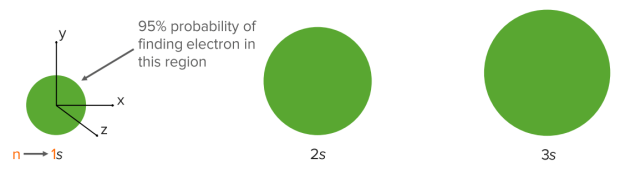1s is an s orbital in shell 1.
2s is an s orbital in shell 2.
Shell 1 (n = 1) has only the 1s orbital.

p Orbitals (I=1) are more complex than s orbitals and have three different types: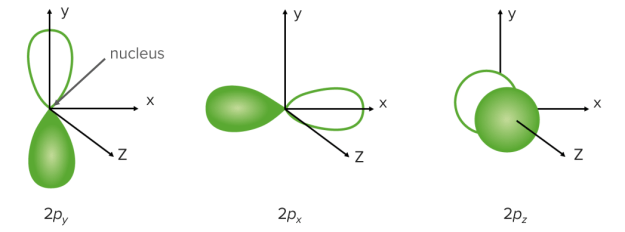Shell 2 (n = 2) has the 2s orbital, which contains 2 electrons, and three 2p orbitals, which contain 6 electrons.

A p orbital has two regions where the electrons may be found on either side of the nucleus:

1.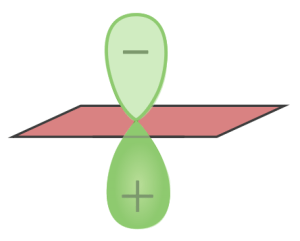Nodal plane
• no electron density
• containing the nucleus
2. Lobes
• are either labeled + or – or given different colors
• said to have different phases

This originates from the wavefunction that defines the orbital: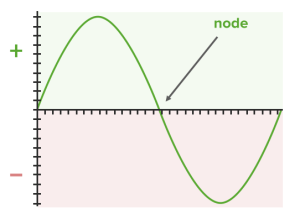Think of a wave with positive and negative regions. In contrast, the d and f orbitals play an important role in the subgroup elements because their partial occupation decisively influences the properties of transition elements (see electron configuration).

Note: The main group and the period uniquely determine the location of each element in the periodic table.

Metals can be found on the left at the bottom of the periodic table; nonmetals are on the right at the top.

## Quantum Numbers

In 1869, Mendeleev developed the law of periodicity based on the regular repetition of similar characteristics of elements. The periodicity described by him is based on the internal structure of atoms and their electron shells in particular.

The periods and the main groups reflect the following rules:

• Atomic radius: equals half the distance between the nuclei of adjacent, identical atoms; within a period, the atomic radii of the main group elements decrease as the atomic number increases because electrons are pulled closer towards the nucleus by the higher number of positively charged protons in the nucleus. Within a group (from the light to the heavy elements), the atomic radii increase as the number of occupied energy levels (shells) increases.
• Mass: the mass increases within the main groups from top to bottom and within the periods from left to right, which correlates with the increasing number of subatomic particles inside the atom.
• Electronegativity: a measure of the tendency of an atom to tighten the bonding electron pair in an atomic compound; the electronegativity of elements increases within a period as the number of protons and the effective nuclear charge rises.

This means that, in a connection between different atoms, the electronegative partner pulls the electron pair in its direction so that the electron pair is no longer located symmetrically between the participating atoms. The electronegativity decreases in these groups because the effective nuclear charge decreases by shielding of the inner orbitals.

### Subgroup elements

The subgroup elements represent the transition metals. The essential biochemical subgroup elements (e.g., zinc, iron, manganese) are called trace elements and should be periodically taken in small amounts by humans. These are important for the function of metalloenzymes.

### Electron configuration and the orbital model

Atoms can be illustrated in diagrams featuring other characteristics, such as the energy level scheme and the electron configuration.

The electron configuration displays the distribution of electrons in the atomic orbitals. It is specific for each atom.

Note: An orbital is a region within the atom where the electrons are highly likely to be found. Each orbital contains a maximum of 2 electrons.

The electron configuration is established by listing each orbital. The following factors must be considered:

Is it a main element or a subgroup element?

→ For main group elements, the last occupied orbital is an s orbital or a p orbital.

→ For subgroup elements, the last occupied orbital is a d orbital or an f orbital.

In which period is the atom?

→ The highest level corresponds to the period in which the atom is located.

Atomic number

→ The number of electrons in a neutral atom equals the atomic number.

1. Aufbau principle: The structure is based on the energetic order, beginning with the lowest energy orbital (s orbital).
2. Pauli principle: An orbital contains a maximum of 2 electrons.
3. Hund’s rule: Orbitals of the same energy are filled individually.

Energy levels/orbitals are stable when they are full, empty, or half full.

Looking at the electron configuration also will tell you the oxidation number of the atom.

### Oxidation numbers

The oxidation number represents the amount of electrons that have been accepted or released by an atom compared to its neutral elemental state.

The oxidation number indicates the ionic charge (valence).

The goal of each atom is to reach a noble gas configuration. To achieve this, the atoms will either release or accept electrons.

Note: The noble gas configuration is an energetically stable arrangement of electrons in which the outer shell is fully occupied with electrons (octet rule). The atoms of all elements try to achieve this state. It has been achieved by the elements of the 8th main group (noble gases), which is why they are inert.

To determine the oxidation number of an atom in a substance, a number of principles and rules must be observed:

1. Pure elements have an oxidation state of zero.
2. Metals have high tendency to release electrons and so they are positively charged.
3. Fluorine: -1
4. Hydrogen: +1
5. Oxygen: -2
6. Halogens: -1

Evidence: If several of the atoms given in this list occur at once, the oxidation numbers are listed according to this hierarchy.

Example: H2O2 → H: +1; O: -1 (the oxidation number of hydrogens stands in the hierarchy above the oxidation number of oxygen; therefore, hydrogen receives its oxidation number as given in the list above)

General determinations

1. Compounds to the outside ± 0
2. Ions outwardly according to their ionic charge

## Chemical Bonding

Here you can find everything concerning chemical bonding.

## States of Matter and Phase Changes

### States of matter

A substance can be solid, liquid, or gas.

• Solid (s): The molecules of a substance are found in a crystal-like structure.
• Liquid (l): The molecules are held together by the forces of attraction but are mutually movable.
• Gaseous (g): The molecules are relatively far apart from each other so that mutual interactions are low.

### Phase changes

This means the change of a state of matter.

During a change in the state of matter, two phases simultaneously coexist (e.g., ice-water mixture, water-steam).

Energy is always consumed during a change of state. If energy is supplied during a phase change in one direction, energy will be released during a change in the other direction.

The following common terms should be known:

solid → liquid: melting

liquid → solid: freezing

liquid → gaseous: vaporizing

gaseous → liquid: condensing

solid → gaseous: sublimating

gaseous → solid: precipitating

## Acids, Bases, & Buffers: The pH Scale and pH Homeostasis

### The pH scale

The pH scale is a measure of hydrogen ion (H+) concentration, and it is a logarithmic scale.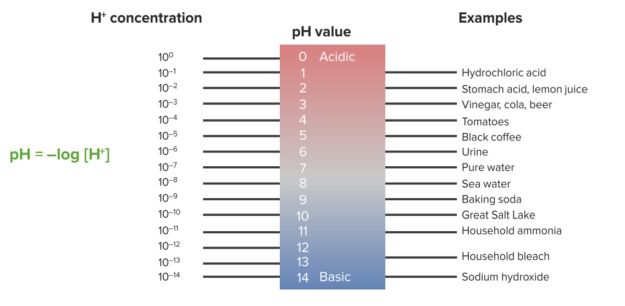Water dissociates into H+ and OH in equal amounts and thus is neutral.

• Acidity: a measure of [H+]
• Basicity: a measure of [OH]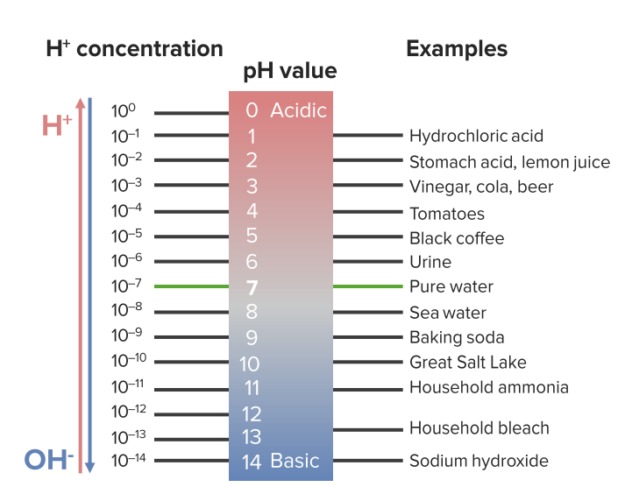### pH Homeostasis

Buffers help cells maintain pH homeostasis.

• Acid: any substance that increases [H+]
• Base: any substance that increases [OH]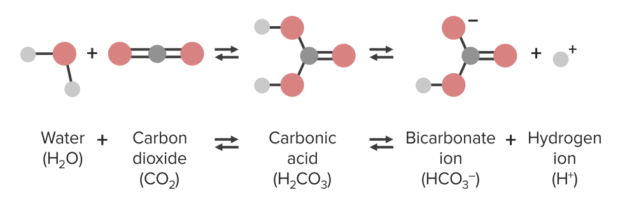• Buffer: anything that resists pH change by binding or releasing H+ ions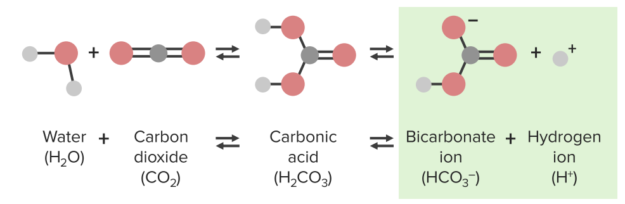## Chemical Reactions

Chemical substances react while forming or dissolving bonds. If forward and reverse reactions plateau after a certain time, there will be a balance of products and reagents.

Important reaction types in inorganic systems are the redox reaction and the acid-base reaction.

### Acid-base reactions

Note: Bronsted acids are compounds that can donate a proton.

On the other hand, a Bronsted base can accept protons, which means it acts as a proton acceptor.

Example:

• NaOH is a base: in an aqueous solution, it dissociates into Na+ and OH. The hydroxide ions are taken by hydrogen ions and water accrues.
• HCl is an acid: in an aqueous solution, HCl reacts with water to produce hydronium and chloride ions.
HCl(aq)  + H2O(l)     → H3O+(aq)  + Cl(aq)
• According to this model, an acid evolves a proton, which means that an oxygen molecule accepts H+ and forms a hydronium ion (H3O+) as a product of the reaction. HCl and Cl represent in this reaction the conjugate acid-base pair 1, and H2O and H3O+  represent the conjugate acid-base pair 2.

A special form of the acid-base reaction is neutralization. Neutralization is a chemical reaction in which hydrogen ions and hydroxide ions combine with each other to form water.

Word equation: hydroxide + acid → salt + water

Note: It is often assumed that an equimolar amount of acids and bases automatically react with each other and form a neutral solution. However, not all of these types of reactions produce a neutral solution. This is only true for strong acid and strong base reactions. If you react a strong acid with a weak partner, an acidic solution will be produced. If you react a strong base with a weak partner, a basic solution will be produced.

### Redox reactions

The redox reaction is a reaction with electron transfer in which oxidation and reduction take place with each other as a partial reaction.  During oxidation, atoms donate electrons, leading to a more positive oxidation state. During reduction, atoms accept electrons, leading to a more negative oxidation state.

The precondition for a redox reaction is the presence of two corresponding electron pairs.

Example: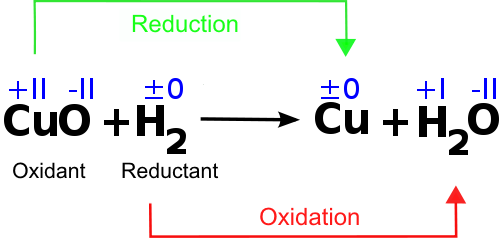As shown in the example, CuO is reduced and afterward called an oxidant. Furthermore, it is shown that the oxidation number of copper changes, while the oxidation number of oxygen stays the same. This means that the atom of copper is the part of the copper oxide-compound that accepts electrons and therefore is involved in the electron transfer.

H2 is oxidized, which means that the electrons will be released, and it acts as a reductant.

Summary: The acid-base-reaction, as well as the redox reaction, are donor-acceptor reactions.  The difference is that, in acid-base-reactions, protons are transferred; in redox reactions, electrons are transferred.

## Quantitative Analysis (Chemical Calculation)

### SI Units

The basic requirement for all chemical calculations is knowledge of essential measurements and fundamental units, which are known as SI units (Système International d’unités).

Unit of atomic mass, u: atomic mass is often expressed in units of daltons (Da)

Amount of substance, n: 1 mol = 6 × 1023 → n = m/M

Mass, m: 1 kg

Molar mass, M: 1 g/mol → M = m/n

Substance concentration, c: 1 mol/cm3

Volume, V: 1 m³

### Law of mass action (LMA)

The LMA provides the mathematical basis for calculating the ratio between products and reactants in an adjusted balance based on the prevailing substance concentration. The ratio K is constant for a specific reaction with the same conditions.

Assumptions:

• Only valid for closed systems
• Only valid for selected equilibria

K = equilibrium/ mass action constant

Stoichiometric numbers of reaction numbers must be considered as an exponent in the LMA.

### Calorimetry

Calorimetry deals with measurements of the released or absorbed heat quantities of chemical reactions.

Assumptions:

• A high rate of reaction
• A complete sequence of chemical reactions
• Measurable heat turnover

Equation for calorimetric calculations:

[cp H2O = 4.19 kJ × kg-1 × K-1]

[RP = reaction product]

### Hess theorem

This theorem states that the reaction enthalpy depends only on the initial and final condition and is independent of the reaction pathway.

If it is not possible to measure the reaction enthalpy directly, it is feasible to determine it indirectly by using the Hess theorem. For this method, the enthalpies of the chemical reactions can be calculated by the listed enthalpy of formation and enthalpy of combustion.

Note: The molar reaction enthalpy is the converted energy of a chemical reaction, based on the amount of substance (unit: kJ/mol).

pH-value calculation

The pH value is the negative decadic logarithm of the hydronium concentration (at 25°C) and therefore is a measure of how acidic or basic a solution is.

This calculation is particularly relevant for acid-base reactions.

Example:

Given: 0.2 mol/L HCl solution.

Since HCl is a strong acid, the concentration of the acid is equal to the concentration of H+.

pH = −log [H+] = −log [0.2] = 0.7

→ 0.7 < 7 (this means that it is a highly acidic solution).

Learn. Apply. Retain.
Your path to achieve medical excellence.
Study for medical school and boards with Lecturio.(Votes: 50, average: 4.46)Loading...